﻿ Scientific & Academic Publishing: The article detailed informationInternational Journal of Optoelectronic Engineering

2012;  2(2): 5-11

doi: 10.5923/j.ijoe.20120202.02

### Optical and Other Measurement Techniques of Carrier Lifetime in Semiconductors

Yeasir Arafat , Farseem M. Mohammedy , M. M. Shahidul Hassan

Department of EEE, BUET, Dhaka, 1000, Bangladesh

Correspondence to: Yeasir Arafat , Department of EEE, BUET, Dhaka, 1000, Bangladesh.
 Email: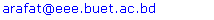Abstract

### 1. Introduction

With the decrease in feature size in semiconductor manufacturing, molecular contamination problems are increased significantly. Recombination lifetime as well as diffusion length measurements have become ubiquitous in the semiconductor industry, because they are a good indicator of wafer contamination. When the defect densities in semiconductors are low, lifetime is one of few parameters that gives significant information about the lower concentration. No other technique can detect defect densities as low as 109–1011 cm−3 in a simple, contactless room temperature measurement. In principle, there is no lower limit to the defect density determined by lifetime measurements. It is for these reasons that the IC industry, largely concerned with unipolar MOS devices in which lifetime plays a minor role, has adopted lifetime measurements as a “process cleanliness monitor”. Alongside this, recombination and generation lifetime as well as measurements of carrier diffusion lengths in semiconductors are also very crucial for photovoltaic devices which have recently become very attractive topic to researchers.
The theory of electron-hole pair recombination through recombination centers (traps) was put forth in 50s of the last century in the well-known works by Shockley-Read-Hall (SRH)[6,7]. Here, we discuss lifetimes, their dependence on semiconductor material and device parameters like energy level, injection level & surfaces and how lifetimes are measured. Different measurement methods can give widely differing lifetimes for the same material or device. In most cases, the reasons for these discrepancies are fundamental and are not due to a deficiency of the measurement. The difficulty with defining a lifetime is that we are describing a property of a carrier within the semiconductor rather than the property of the semiconductor itself.

### 2. Theory

There are two principal categories of carrier lifetimes: recombination lifetimes and generation lifetimes. The concept of recombination lifetime τr holds when excess carriers decay as a result of recombination. Generation lifetime τg applies when there is a paucity of carriers, as in the space- charge region of a reverse-biased device and the device tries to attain equilibrium. During recombination an electron-hole pair ceases to exist on average after a time τr, illustrated in Fig. 1(a). The generation lifetime, by analogy, is the time that it takes on average to generate an ehp, illustrated in Fig. 1(b). Thus generation lifetime is a misnomer, since the creation of an ehp is measured and generation time would be more appropriate. Nevertheless, the term “generation lifetime” is commonly accepted. When these recombination and generation events occur in the bulk, they are characterized by τr and τg. When they occur at the surface, they are characterized by the surface recombination velocity sr and the surface generation velocity sg, also illustrated in Fig. 1. Both bulk and surface recombination or generation occur simultaneously and their separation is sometimes quite difficult. Consisting of bulk and surface components, the measured lifetimes are always effective lifetimes.
It is instructive to consider τr and τg in more detail before discussing lifetime measurement techniques. The excess ehps may have been generated by photons or particles of energy higher than the band gap or by forward biasing a pn junction. There are more carriers after the stimulus than before and the excess carriers return to equilibrium by recombination.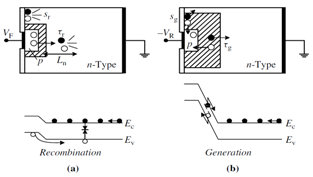Figure 1. Illustration of various recombination and generation mechanisms for a (a) forward-biased and (b) reverse-biased junction

#### 2.1. Recombination Lifetime and Surface Velocity

The departure of the carrier densities from their equilibrium values non-linearly controls the bulk recombination rate R. We consider a p-type semiconductor throughout this work and are chiefly concerned with the behavior of the minority electrons. Confining ourselves to linear, quadratic, and third order terms, R can be written as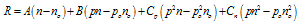(1)
where n = no + Δn, p = po + Δp, no, po are the equilibrium and Δn, Δp, the excess carrier densities. In the absence of trapping, Δn = Δp, allowing Eq. (1) to be simplified to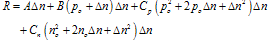(2)
where some terms containing no have been dropped because no << po in a p-type material. The recombination lifetime is defined as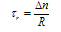(3a)
giving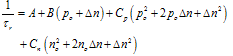(3b)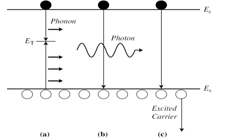Figure 2. Variuos mechanisms: (a) SRH (b) radiative and (c) Auger
There are three main recombination mechanisms those determine the recombination lifetime: SRH or multiphonon recombination characterized by τSRH , radiative recombination characterized by τrad and Auger recombination characterized by τAuger. These mechanisms are illustrated in Fig. 2.
The recombination lifetime τr is determined according to the relationship given below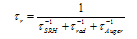(4)
1) SRH Recombination: During SRH recombination, ehps recombine through deep-level impurities or traps, characterized by the density NT, energy level ET and capture cross-sections σn and σp for electrons and holes, respectively. The energy liberated during the recombination event is dissipated by lattice vibrations or phonons, illustrated in Fig. 2(a). The SRH lifetime is given by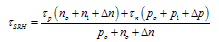(5)
where n1, p1, τn and τp are defined as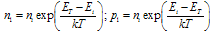(6a)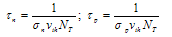(6b)
2) Radiative Recombination: During this recombination, ehps recombine directly from band to band with the energy carried away by photons as shown in Fig. 2(b). The radiative lifetime is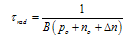(7)
B is the radiative recombination coefficient. The radiative lifetime is inversely proportional to the carrier density because in band-to-band recombination both electrons and holes must be present simultaneously.
3) Auger recombination: During Auger recombination, as illustrated in Fig. 2(c), the recombination energy is absorbed by a third carrier and the Auger lifetime is inversely proportional to the carrier density squared. The Auger lifetime is given by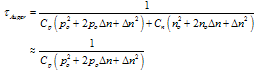(8)
where Cp is the Auger recombination coefficient for holes and Cn for electrons. Values for radiative and Auger coefficients are given in Table 1.
 Table 1. Recombination Coefficients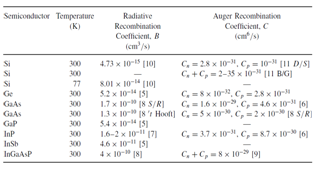#### 2.2. Recombination Lifetime and Level of Injections

Equations (5) to (8) simplify for both low-level and high-level injection. Low-level injection holds when the excess minority carrier density is low compared to the equilibrium majority carrier density, Δn<<po. Similarly, high- level injection holds when Δn>>po. The injection level is important during lifetime measurements. The appropriate expressions for low-level (ll) and for high-level (hl) injection become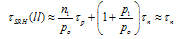(9)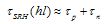(9a)
where the second approximation in the τSRH(ll) expression holds when n1<<po and p1<<po.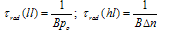(10)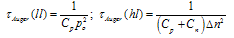(11)
The Si recombination lifetimes according to Eq. (4) are plotted in Fig. 3. At high carrier densities, the lifetime is controlled by Auger recombination and at low densities by SRH recombination. Auger recombination has the characteristic 1/n2 dependence. The high carrier densities may be due to high doping densities or high excess carrier densities.
Whereas SRH recombination is controlled by the cleanliness of the material, Auger recombination is an intrinsic property of the semiconductor. Radiative recombination plays almost no role in Si except for very high lifetime substrates but is important in direct band gap semiconductors like GaAs.
The bulk SRH recombination rate is given by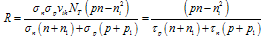(12)
The surface SRH recombination rate is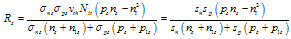(13)
Where sn = σnsvthNit; sp = σpsvthNit. The subscript “s” refers to the appropriate quantity at the surface; ps and ns are the hole and electron densities (cm−3) at the surface. The interface trap density Nit (cm−2) is assumed constant in Eq. (13). If not constant, the interface trap density Dit (cm−2 eV−1) must be integrated over energy with Nit in these equations given by Nit≈ kTDit.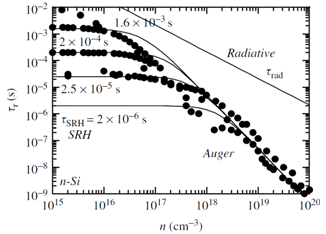Figure 3. Recombination lifetime versus majority carrier density for n-Si with Cn = 2 × 10−31 cm6/s and B = 4.73 × 10−15 cm3/s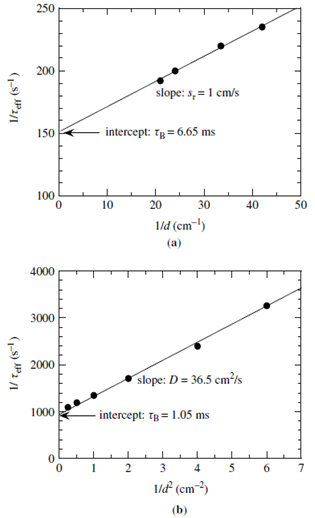Figure 4. Determination of bulk lifetime, surface recombination velocity and diffusion coefficient from lifetime measurements. Data from 
The surface recombination velocity sr is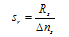(14a)
from eq. (13)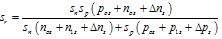(14b)
The surface recombination velocity for low-level and high-level injection becomes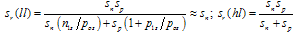(15)
sr depends strongly on injection level for the SiO2/Si interface as shown in Fig. 4.

#### 2.3. Recombination Lifetime Measurements Techniques

Recombination lifetime can be measured optically or electrically. The commonly used techniques are
1) Optical Measurements: Photoconductance Decay (PCD), Quasi-Steady-State Photoconductance (QSSPC), Short-Circuit Current/Open-Circuit Voltage Decay (SCCD/ OCVD), Photoluminescence Decay (PLD), Surface Photovoltage (SPV), Steady-State Short-Circuit Current (SSSCC), Free Carrier Absorption, Electron Beam Induced Current (EBIC) etc.
2) Electrical Measurements: Diode Current-Voltage, Reverse Recovery (RR), Open-Circuit Voltage Decay (OCVD), Pulsed MOS Capacitor, Other Techniques.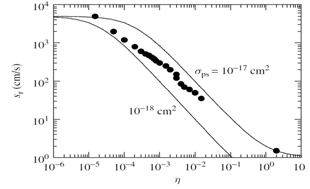Figure 5. The surface recombination velocity sr versus injection level η as a function of σps for Nit = 1010 cm−2, pos = 1016 cm−3, ETs = 0.4 eV, σns = 5 × 10−14 cm2. Data from 

#### 2.4. Generation Lifetime and Surface Velocity

Every recombination process of Fig. 2 has a generation counterpart. The inverse of multiphonon recombination is thermal ehp generation in Fig. 1(b). The inverse of radiative and Auger recombination are optical and impact ionization generation. Optical generation is negligible for a device in the dark and with negligible blackbody radiation from its surroundings. Impact ionization is usually considered to be negligible for devices biased sufficiently below breakdown voltage. However, impact ionization at low ionization rates can occur at low voltages and care must be taken to eliminate this generation mechanism during τg measurements.
Generation dominates for pn < n2i. Furthermore the smaller the pn product, the higher is the generation rate. R becomes negative and is then designated as the bulk generation rate G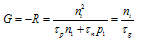(16)
for pn ≈ 0 with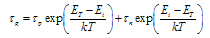(17)
The condition pn→0 is approximated in the scr of a reverse-biased junction.
The quantity τg, defined in Eq. (17), is the generation lifetime that depends inversely on the impurity density and on the capture cross-section for electrons and holes, just as recombination does. The generation lifetime can be quite high if ET does not coincide with Ei. Generally, τg is higher than τr, at least for Si devices, where detailed comparisons have been made and τg ≈ (50–100)τr.
When psns < n2i at the surface, we find from Eq. (13), the surface generation rate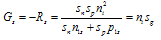(18)
where sg is the surface generation velocity, given by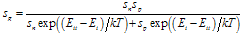(19)
From Eqs. (14b) and (19), for EitEi it is found that sr > sg.
Generation lifetime is usually measured by electrical means such as Gate-Controlled Diode and Pulsed MOS Capacitor methods.

### 3. Methodology

Of the various lifetime measurement methods, optical technique is stated in the following section.

#### 3.1. Recombination Lifetime: Optical Measurements

Consider a p-type semiconductor with light incident on the sample. The light may be steady state or transient. The continuity equation for uniform ehp generation and zero surface recombination is [12,13]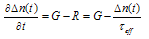(20)
where Δn(t) is the time dependent excess minority carrier density, G the ehp generation rate and τeff the effective lifetime. Solving for τeff gives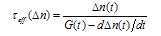(21)
In the transient PCD method, with G(t) << dΔn(t)/dt, the effective lifetime becomes τeffn) = -Δn(t)/. In the steady-state method, with G(t) >> dΔn(t)/dt, the effective lifetime becomes τeffn) = Δn/G and in the QSSPC method, Eq. (21) remains valid. Both Δn and G need to be known in the steady-state and QSSPC methods to determine the effective lifetime.
The excess carrier density decay for low level injection is given by Δn(t) = Δn(0)exp(-t/τeff) where τeff is calculated as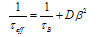(22)
with β found from the relationship tan(βd/2) = sr/(βD). Here τB is the bulk recombination lifetime, D the minority carrier diffusion constant under low injection level and the ambipolar diffusion constant under high injection level and d the sample thickness. Equation (22) holds for any optical absorption depth provided the excess carrier density has ample time to distribute uniformly, i.e., d << (Dt)1/2. The effective lifetime of Eq. (20) is plotted in Fig. 5 versus d as a function of sr.
For thin samples, τeff no longer bears any resemblance to τB, the bulk lifetime and is dominated by surface recombination. The surface recombination velocity must be known to determine τB unambiguously unless the sample is sufficiently thick. Although the surface recombination velocity of a sample is generally not known, by providing the sample with high sr, by sandblasting for example, it is possible to determine τB directly. However, the sample must be extraordinarily thick. Equation (22) can be written as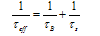(23)
where τs is the surface lifetime.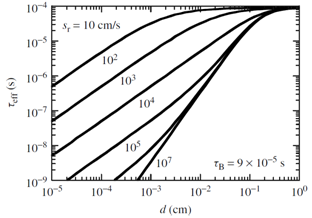Figure 6. Effective lifetime versus wafer thickness as a function of surface recombination velocity. D = 30 cm2/s
Two limiting cases are of particular interest: sr→0 gives tan(βd/2) ≈ βd/2 and sr→∞ gives tan(βd/2)≈∞ or βd/2 ≈ π/2, making the surface lifetime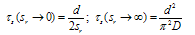(24)
For sr→0, a plot of 1/τeff versus 1/d has a slope of 2sr and an intercept of 1/τB, allowing both sr and τB to be determined. For sr→∞, a plot of 1/τeff versus 1/d2 has a slope of π2D and an intercept of 1/τB. Both examples are illustrated in Fig. 6. The approximation τs = d/2sr holds for sr < D/4d.
Equations (22)–(24) hold for samples with one dimension much smaller than the other two dimensions, for example, a wafer. For samples with none of the three dimensions very large, Eq. (23 becomes for sr→∞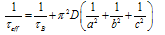(25)
where a, b and c are the sample dimensions. It is recommended that the sample surfaces have high surface recombination velocities. The recommended dimensions and the maximum bulk lifetimes that can be determined through Eq. (23) for Si samples are given in Table 2.
 Table 2. Recommended Dimensions for PCD Samples and Maximum Bulk Lifetimes for Silicon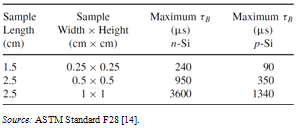1) Photoconductance Decay: The photoconductance decay lifetime characterization technique was proposed in 1955  and has become one of the most common lifetime measurement techniques. As the name implies, ehps are created by optical excitation and their decay is monitored as a function of time following the cessation of the excitation. Other excitation means such as high-energy electrons and gamma rays can also be used. The samples may either be contactless (Fig. 7) or the measurement can be contacted with the current being monitored (Fig. 8).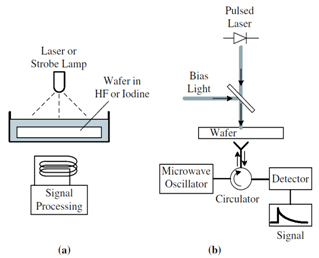Figure 7. PCD measurement schematic for contactless (a) rf bridge and (b) microwave reflectance measurements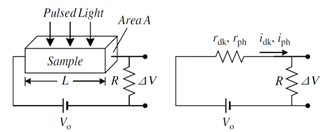Figure 8. Schematic diagram for contact photoconductance decay measurement
2) Quasi-Steady-State Photoconductance (QSSPC): In the QSSPC method the sample is illuminated with a flash lamp with a decay time constant of several ms and an illumination area of several cm2[7,9]. Due to the slow decay time, the sample is under quasi steady-state conditions during the measurement as the light intensity varies from its maximum to zero. The steady-state condition is maintained as long as the flash lamp time constant is longer than the effective carrier lifetime. The timevarying photoconductance is detected by inductive coupling. The excess carrier density is calculated from the photoconductance signal. The generation rate, required in Eq. (7.25), is determined from the light intensity measured with a calibrated detector. Semiconductors absorb only a fraction of the incident photons, depending on the reflectivity of the front and back surfaces, possible faceting of those surfaces, and the thickness of the wafer. The value of the absorption fraction for a polished, bare silicon wafer is f ≈ 0.6.
If the wafer has an optimized antireflection coating, f ≈ 0.9, while a textured wafer with antireflection coating can approach f ≈ 1.36 The generation rate per unit volume G can then be evaluated from the incident photon flux and the wafer thickness, according to where is the photon flux density and the sample thickness. Assuming the flash lamp light decay is exponential in time, the generation rate is higher for τeff < τflash, the sample is in quasi steady-state during the measurement. Hence, the flash lamp decay time must be sufficiently long for the QSSCP measurement to be valid.

### 4. Conclusions

The microwave reflection or inductive coupling photoconductance decay technique is commonly used to measure carrier lifetime. Its key strength is the contactless nature and rapidness and major weakness is the unknown surface recombination velocity. If the sample thickness can be changed, then both the bulk lifetime and the surface recombination velocity can be extracted. The quasi-steady-state photoconductance method is a more recent method and has found wide acceptance in the design of photovoltaic devices. It measures the lifetime as a function of injection level in one step but requires large sample area (several cm2) precluding high density mapping. Surface photovoltage technique is used to detect iron in p-Si. The most common electrical recombination lifetime method is the open-circuit voltage decay method. Measured τr or Ln mean little for thin layers, e.g., epitaxial layers on highly doped substrates, denuded zones on heavily precipitated substrates, or SOI films. Such layers are best characterized through generation lifetime characterization  which is commonly determined with the pulsed MOS capacitor. The Zerbst plot implementation is the most common, but the current versus inverse capacitance is easier to interpret because the doping density of the sample need not be known. Since τg is measured in the space-charge region of a reverse-biased device, it lends itself easily for the characterization of thin layers. Since the scr width can be varied by an applied voltage, it is possible to generate a τg depth profile, that is difficult to do with τr measurements, because the measurement depth for τr and Ln measurements is the minority carrier diffusion length.

### ACKNOWLEDGEMENTS

Authors of this paper would like to thank the Department of EEE, BUET, Dhaka-1000, Bangladesh, for its various supports during the preparation of this manuscript.

### References

  U.B. Godse, Simulations of removal of molecular contaminants from silicon wafer surface, PhD Thesis, UT Austin, December 2011.  P.Y. Yu, M. Cardona, Fundamentals of Semiconductors: Physics and Materials Properties, 4th ed., Springer, 2010.  S.M. Sze, K.K. Ng, Physics of semiconductor devices, 3rd ed., John Wiley & Sons, 2007.  D. K. Schroder, Semiconductor Material and Device Characterization, 3rd ed., John Wiley & Sons, Inc., Hoboken, New Jersey, USA, 2006.  M.C. Putnam, D.B.T. Evans, M.D. Kelzenberg, et al. “10 μm minority-carrier diffusion lengths in Si wires synthesized by Cu-catalyzed vapor-liquid-solid growth,” Appl. Phys. Lett., vol. 95, 2009.  W. Shockley and W.T. Read, “Statistics of the Recombinations of Holes and Electrons,” Phys.Rev., vol. 87, pp. 835–842, Sept. 1952.  R.N. Hall, “Recombination Processes in Semiconductors,” Proc. IEE, vol. 106B, pp. 923–931, March, 1960.  Y.P. Varshni, “Band-to-Band Radiative Recombination in Groups IV, VI and III-V Semiconductors (I) and (II),” Phys. Stat. Sol., vol. 19, pp. 459–514, Feb. 1967; ibid. vol. 20, pp. 9–36, March 1967.  D.J. Fitzgerald and A.S. Grove, “Surface Recombination in Semiconductors,” Surf. Sci., vol. 9, pp. 347–369, Feb. 1968.  S.K. Pang and A. Rohatgi, “A New Methodology for Separating Shockley-Read-Hall Lifetime and Auger Recombination Coefficients from the Photoconductivity Decay Technique,” J. Appl. Phys., vol. 74, pp. 5554–5560, Nov. 1993.  A.G. Aberle, S. Glunz, and W. Warta, “Impact of Illumination Level and Oxide Parameters on Shockley-Read-Hall Recombination at the Si-SiO2 Interface,” J. Appl. Phys., vol. 71, pp. 4422–4431, May 1992.  J.I. Pankove, Optical Processes in Semiconductors, 2nd ed., Dover Publications, 2010.  H. Nagel, C. Berge, and A.G. Aberle, “Generalized Analysis of Quasi-Steady-State and Quasi-Transient Measurements of Carrier Lifetimes in Semiconductors,” J. Appl. Phys., vol. 86, pp. 6218–6221, Dec. 1999.  ASTM Standard F28-91, “Standard Method for Measuring the Minority-Carrier Lifetime in Bulk Germanium and Silicon,” 1996.  D.T. Stevenson and R.J. Keyes, “Measurement of Carrier Lifetimes in Germanium and Silicon,” J. Appl. Phys., vol. 26, pp. 190–195, Feb. 1955.  D.K. Schroder, B.D. Choi, S.G. Kang, W. Ohashi, K. Kitahara, G. Opposits, T. Pavelka, and J.L. Benton, “Silicon Epitaxial Layer Recombination and Generation Lifetime Characterization,” IEEE Trans. Electron Dev., vol. 50, pp. 906–912, April 2003.